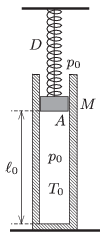Mathematical and Physical Journal
for High Schools
Issued by the MATFUND Foundation
 Already signed up? New to KöMaL?

#Problem P. 4729. (April 2015)

P. 4729. An easily moveable piston of mass $\displaystyle M=80$ kg is suspended by a thread in a vertical cylinder of inner cross section $\displaystyle A=5~{\rm dm}^2$, and encloses a sample of air at a temperature of $\displaystyle T_0=300$ K. The piston is attached to the ceiling by an unstretched spring of spring constant $\displaystyle D=6$ N/cm. The length of the air column is $\displaystyle \ell_0=1.2$ m, and initially the pressure inside and outside is $\displaystyle p_0=10^5$ Pa. At a moment the thread breaks.$\displaystyle a)$ What will the greatest speed of the piston be during the initiated process?

$\displaystyle b)$ During the process what will the greatest temperature of the enclosed sample of air be?

(5 pont)

Deadline expired on May 11, 2015.

### Statistics:

 36 students sent a solution. 5 points: Asztalos Bogdán, Balogh Menyhért, Bekes Nándor, Berta Dénes, Blum Balázs, Bugár 123 Dávid, Csathó Botond, Fehér Balázs, Fekete Panna, Forrai Botond, Fülöp Erik, Holczer András, Jakus Balázs István, Kaposvári Péter, Kasza Bence, Kovács Péter Tamás, Körtefái Dóra, Marosvári Kristóf, Németh Flóra Boróka, Olosz Balázs, Sal Kristóf, Szántó Benedek, Wiandt Péter. 4 points: Molnár Elizabet. 3 points: 5 students. 2 points: 6 students. 1 point: 1 student.

Problems in Physics of KöMaL, April 2015